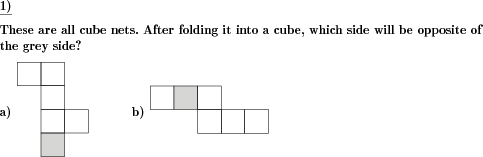Custom math worksheets at your fingertips# Details for problem "Cube net find opposite side of marked side"

Quickname: 6084

Elementary School, Primary School, Middle School.

## Summary

Find the opposite side in a cube net.

## Example## Description

3-D figures are a geometry topic and a foundation for emerging technologies. An interesting and fantastic worksheet to learn about three-dimensional figures. Cube nets are the result of unwinding a cube, i.e. a three-dimensional body. A two-dimensional figure emerges, which consists of squares that are connected at the sides. This figure is called a cube net. Each cube net consists of six squares corresponding to the sides of the cube.

In this exercise, a series of cube nets is presented. In each cube net, a square is marked. When this cube net is folded again to form a cube, this side will have a counterpart on the cube. The task is to find the square opposite to the cube after folding it.

Use these accessible worksheets in groups to have a fun learning experience in grades 3 to 5. Practicing these worksheets enables learners to build up strong basic geometric concepts, some objectives are as follows.

1. Figure out 2-D shapes and 3-D shapes.
2. Recognize the relationship between 2-D and 3-D shapes.
3. Grasp the skill of mapping the opposite side when converting a 2-D shape to a 3-D shape.
4. Strong concepts of 3-D shapes will lay a foundation for cutting-edge technologies.

The number of problems is adjustable.

The answer key will include each 2-D shape shaded for the opposite side.

Download free printable worksheets for this math problem here. The worksheet contains the problems only, the solution sheet includes the answers. Just click on the respective link.

•Worksheet 1Solution sheet with answers
•Worksheet 2Solution sheet with answers
•Worksheet 3Solution sheet with answers

If you can not see the solution sheets for download, they may be filtered out by an ad blocker that you may have installed. If this is the case, please allow ads for this page and reload the page. The solution sheets will then reappear.

• Do these sample worksheets do not really fit?
• Do you need more math worksheets, with a different level of difficulty?
• Would you like to combine different problems on a worksheet and adjust them to your needs?
• As a teacher, you can put together your own worksheets using the automatically generated math problems provided.
With a free initial credit, you can start creating your own math worksheets in a few minutes.

You can try it for free! Register here, to create custom worksheets now!

## Customization options for this problem

Parameter
Possible values
Number of problems
1, 2, 3, 4, 5, 6, 7, 8, 9, 10